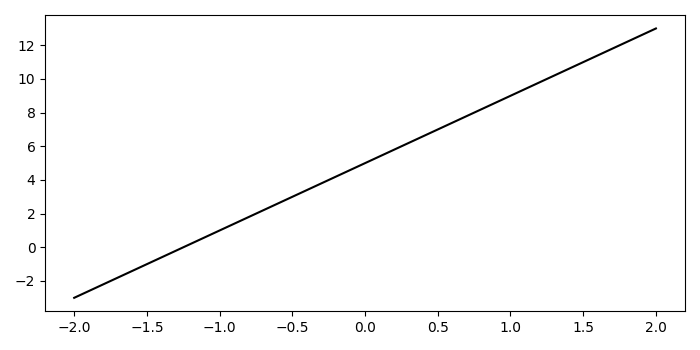# How to change the plot line color from blue to black in Matplotlib?

To change the plot line color from blue to black, we can use setcolor() method−

## Steps

• Create x and y data points using numpy.
• Plot line x and y using plot() method; store the returned value in line.
• Set the color as black using set_color() method.
• To display the figure, use show() method.

## Example

import numpy as np
from matplotlib import pyplot as plt
plt.rcParams["figure.figsize"] = [7.00, 3.50]
plt.rcParams["figure.autolayout"] = True
x = np.linspace(-2, 2, 10)
y = 4 * x + 5
line, = plt.plot(x, y, c='b')
line.set_color('black')
plt.show()

## Output